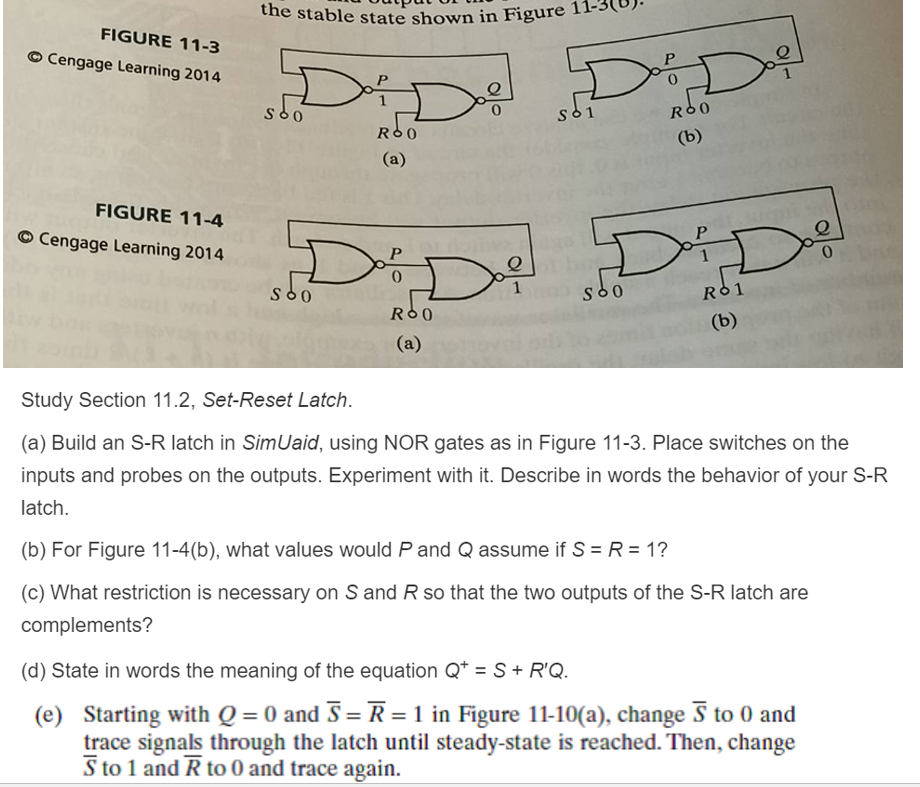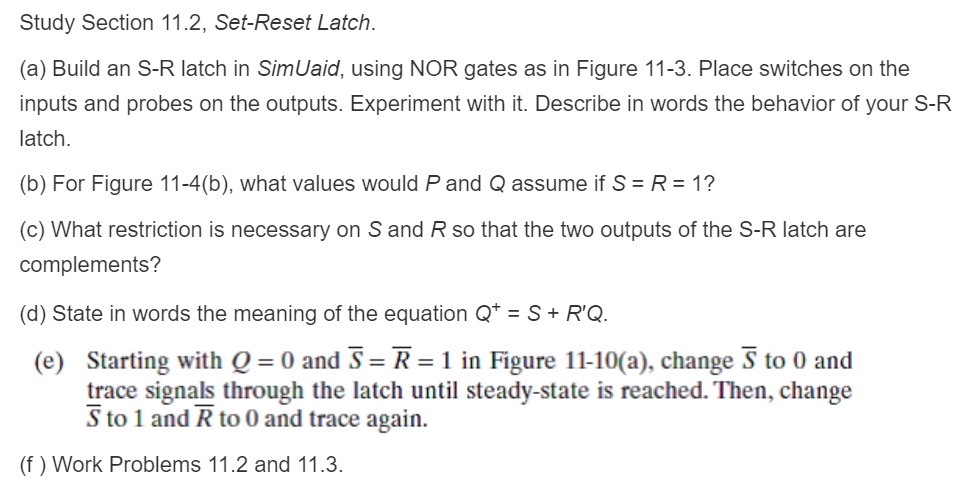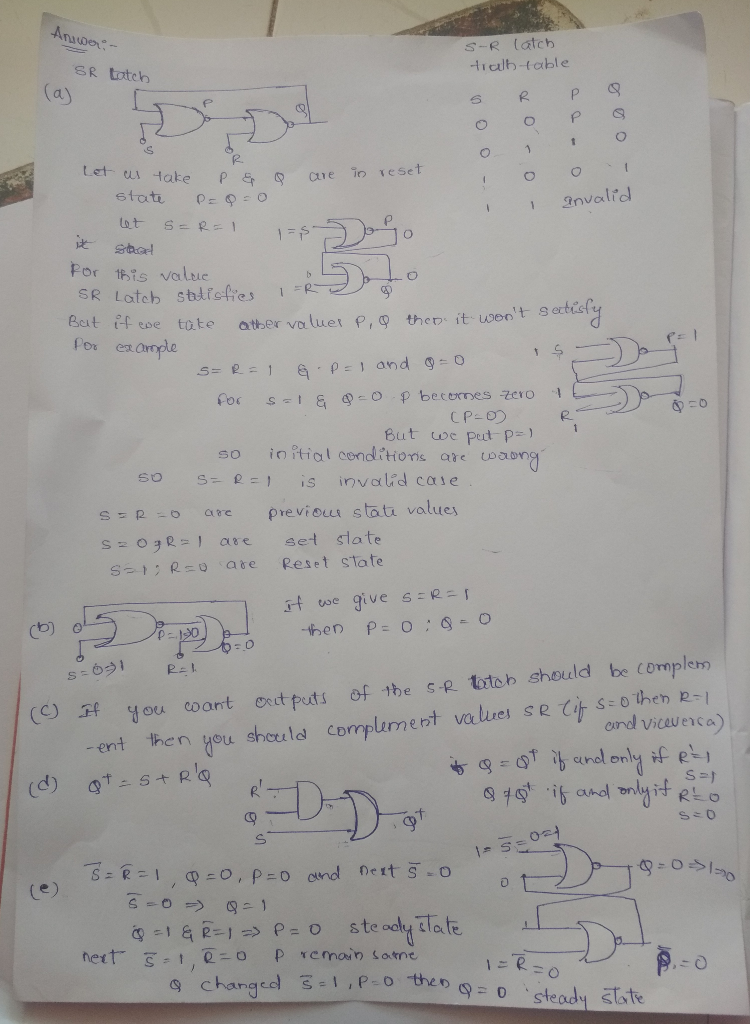# 1-3(0). Figure 1 the stable state shown in FIGURE 11-3 Cengage Learning 2014 0 0 0 RSO FIGURE 11-4 Cengage Learning 201...1-3(0). Figure 1 the stable state shown in FIGURE 11-3 Cengage Learning 2014 0 0 0 RSO FIGURE 11-4 Cengage Learning 2014 0 0 Study Section 11.2, Set-Reset Latch. (a) Build an S-R latch in SimUaid, using NOR gates as in Figure 11-3. Place switches on the inputs and probes on the outputs. Experiment with it. Describe in words the behavior of your S-R latch (b) For Figure 11-4(b), what values would P and Q assume if S = R = 1? (c) What restriction is necessary on S and R so that the two outputs of the S-R latch are complements? (d) State in words the meaning of the equation Q S+R'Q. Starting with Q-0 and 3-R-1 in Figure 11-10(a), change ริ to 0 and (e) trace signals through the latch until steady-state is reached. Then, change S to 1 and R to 0 and trace again.
Study Section 11.2, Set-Reset Latch (a) Build an S-R latch in SimUaid, using NOR gates as in Figure 11-3. Place switches on the inputs and probes on the outputs. Experiment wth t. Describe in words the behavior of your S-R atch (b) For Figure 11-4(b), what values would P and Q assume if SR 1? (c) What restriction is necessary on S and R so that the two outputs of the S-R latch are complements? (d) State in words the meaning of the equation Q SR'Q Starting with Q-0 and s-R-1 in Figure 11-10(a), change ริ to 0 and (e) trace signals through the latch until steady-state is reached. Then, change S to 1 and R to 0 and trace again. (f) Work Problems 11.2 and 11.3.##### Add Answer of: 1-3(0). Figure 1 the stable state shown in FIGURE 11-3 Cengage Learning 2014 0 0 0 RSO FIGURE 11-4 Cengage Learning 201...
More Homework Help Questions Additional questions in this topic.